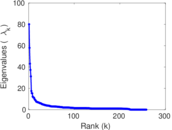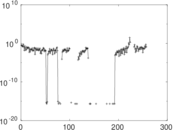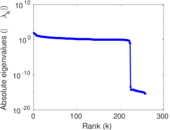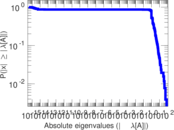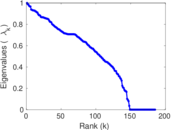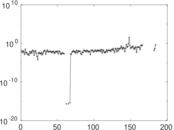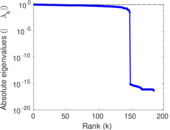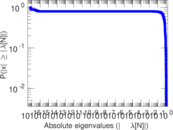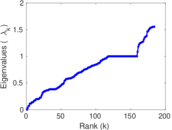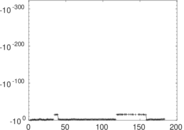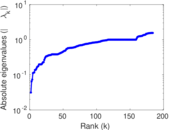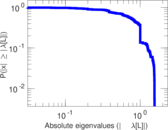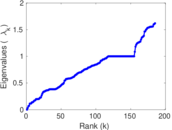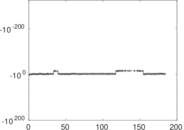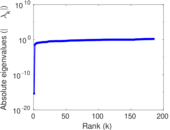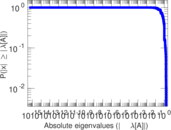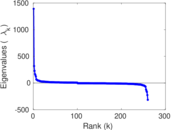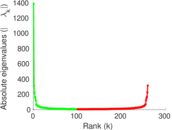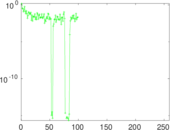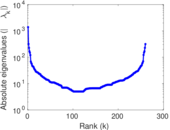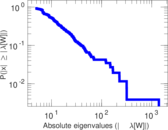# Wikiquote edits (sk)

This is the bipartite edit network of the Slovak Wikisource. It contains users and pages from the Slovak Wikisource, connected by edit events. Each edge represents an edit. The dataset includes the timestamp of each edit.

 Code `qsk` Internal name `edit-skwikisource` Name Wikiquote edits (sk) Data source http://dumps.wikimedia.org/ AvailabilityDataset is available for download Consistency checkDataset passed all tests Category Authorship network Dataset timestamp 2017-10-20 Node meaning User, article Edge meaning Edit Network formatBipartite, undirected Edge typeUnweighted, multiple edges Temporal dataEdges are annotated with timestamps

## Statistics

 Size n = 1,403 Left size n1 = 260 Right size n2 = 1,143 Volume m = 3,143 Unique edge count m̿ = 2,072 Wedge count s = 135,267 Claw count z = 11,210,656 Cross count x = 827,275,393 Square count q = 18,988 4-Tour count T4 = 699,548 Maximum degree dmax = 542 Maximum left degree d1max = 542 Maximum right degree d2max = 154 Average degree d = 4.480 40 Average left degree d1 = 12.088 5 Average right degree d2 = 2.749 78 Fill p = 0.006 972 21 Average edge multiplicity m̃ = 1.516 89 Size of LCC N = 1,225 Diameter δ = 12 50-Percentile effective diameter δ0.5 = 3.734 50 90-Percentile effective diameter δ0.9 = 5.811 96 Median distance δM = 4 Mean distance δm = 4.391 12 Gini coefficient G = 0.698 092 Balanced inequality ratio P = 0.225 899 Left balanced inequality ratio P1 = 0.146 675 Right balanced inequality ratio P2 = 0.317 849 Relative edge distribution entropy Her = 0.826 154 Power law exponent γ = 3.061 35 Tail power law exponent γt = 2.681 00 Tail power law exponent with p γ3 = 2.681 00 p-value p = 0.002 000 00 Left tail power law exponent with p γ3,1 = 1.831 00 Left p-value p1 = 0.470 000 Right tail power law exponent with p γ3,2 = 3.681 00 Right p-value p2 = 0.123 000 Degree assortativity ρ = −0.134 090 Degree assortativity p-value pρ = 8.917 51 × 10−10 Spectral norm α = 80.035 6 Algebraic connectivity a = 0.031 283 2 Spectral separation |λ1[A] / λ2[A]| = 1.380 04 Controllability C = 952 Relative controllability Cr = 0.680 973

## Plots

### Fruchterman–Reingold graph drawing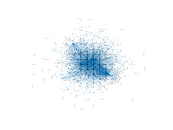### Degree distribution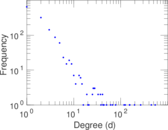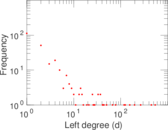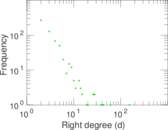### Cumulative degree distribution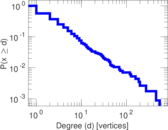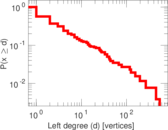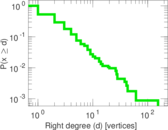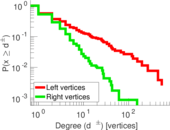### Lorenz curve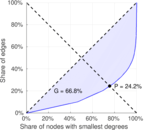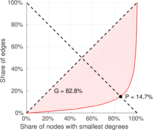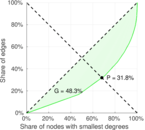### Spectral distribution of the adjacency matrix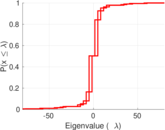### Spectral distribution of the normalized adjacency matrix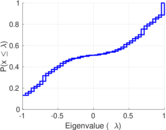### Spectral distribution of the Laplacian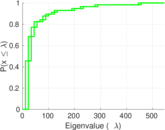### Spectral graph drawing based on the adjacency matrix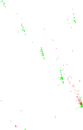### Spectral graph drawing based on the Laplacian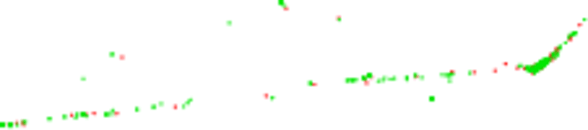### Spectral graph drawing based on the normalized adjacency matrix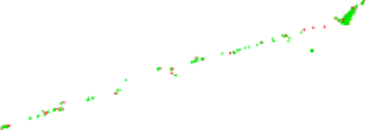### Degree assortativity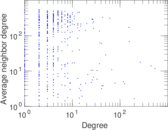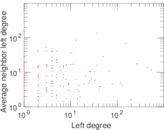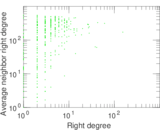### Zipf plot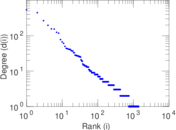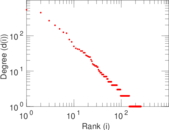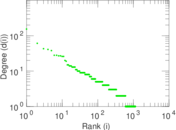### Hop distribution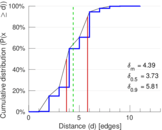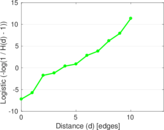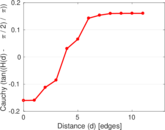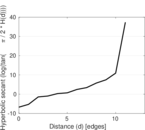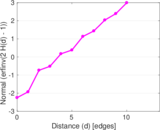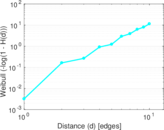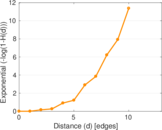### Double Laplacian graph drawing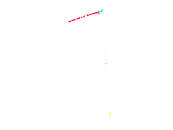### Delaunay graph drawing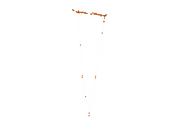### Edge weight/multiplicity distribution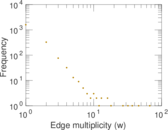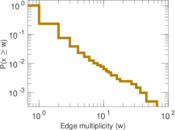### Temporal distribution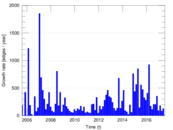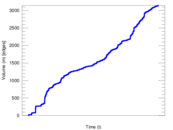### Temporal hop distribution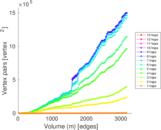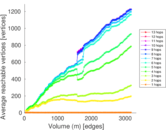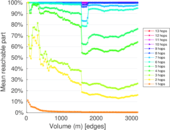### Diameter/density evolution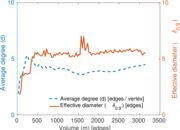### Matrix decompositions plots